Right Triangles
edHelper subscribers - Create a new printable

Number of Keys
 Select the number of different printables: 1 key 2 keys 3 keys 4 keys 5 keys

Number of Pages (for each key)
 Select the number of pages: 1 page 2 pages 3 pages 4 pages

Include an answer key (answer keys will be at the end of the printable)
High School Geometry
High School Geometry
Math

 Name _____________________________Date ___________________ (Key 1 - Answer ID # 0428216)
1.

If PQ = 12, RS = 9, and PS = 10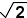, find QR.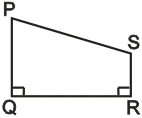2.

In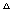ABC, AB = x, AC = 2x, and BC = 5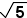. Find the value of x.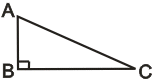3.

A rectangle has a diagonal of 10 and length of 4. Find the area of the rectangle.

4.

Find the length of the diagonal of a rectangle with sides 14 cm and 14cm.

5.

The bases of isosceles trapezoid ABCD are 16 cm and 20 cm and its base angles (angles A and B) are 45 degrees. What is the height of the trapezoid?

6.

The lengths of the bases of an isosceles trapezoid are 40 and 16, and the length of the altitude is 9. Find the length of a leg of the trapezoid.

7.

If s = 19 and t = 21, then r = ______.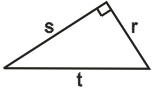8.

If a = 15 and b = 11, find c.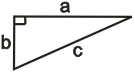9.

Find the length of the altitude to the base of an isosceles triangle with sides 11, 11, and 16.

10.

The length of the hypotenuse of a right isosceles triangle is 4. What is the perimeter of the triangle?

Right Triangles
edHelper subscribers - Create a new printable

Number of Keys
 Select the number of different printables: 1 key 2 keys 3 keys 4 keys 5 keys

Number of Pages (for each key)
 Select the number of pages: 1 page 2 pages 3 pages 4 pages